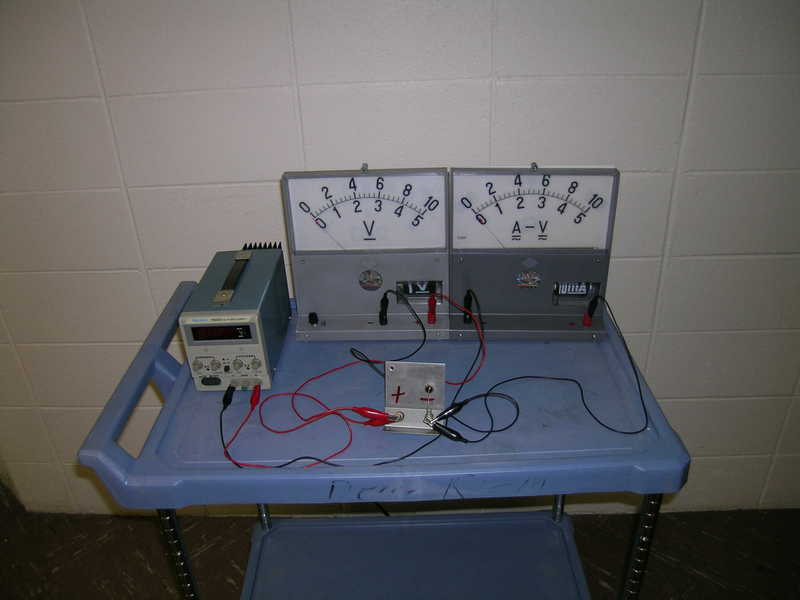# SEMICONDUCTOR

5M10.10 HALL EFFECT
5M10.50 DIODE

 5M10.10 Hall Effect The Hall Effect probe will produce a voltage proportional to the strength of the magnetic field.Setup Requirements: Assembled as needed. Ask at least 1 day  ahead of time.   Equations: E(z) = v(x) B(y) and  V(Hall) = R I B/ t. R is Hall coefficient and t is thickness.    Safety Issues: Strong heavy magnet

 5M10.50 Diode (Variable R) Construct circuit and collect data to show diode does not follow Ohm's Law.Setup Requirements: Assembled as needed. Ask at least 1 day ahead of time.   Equations:I does not = V/R with fixed R. I = A(e^qV/kT-1). I proportional to V for small values of qV/kt but increases exponentially as V increases.   Safety Issues: Limit current and voltage used to avoid damaging diode.